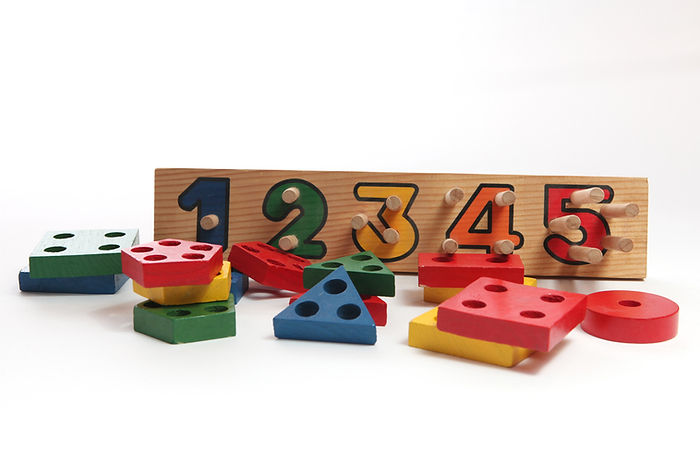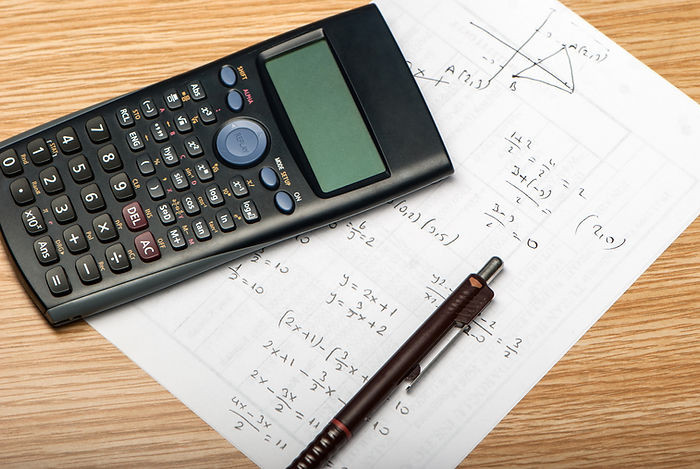Math Bingo @4PM

## CHECK IT OUT AND HAVE SOME FUN!

Below are links to the Math Bingo game cards. There are four cards ranging from first grade through middle school math. Please select the level you feel most comfortable. You will need to complete the Bingo card PRIOR to attending as the answers will be called out and we do quick math! So come have some fun with us a win a few prizes!Single, double and triple digit addition and subtraction### MULTIPLICATION/DIVISION

Single, double and triple multiplication, simple and long division problems### FRACTIONS

Addition, subtraction, multiplication, and division of fractions### ALGEBRAIC EQUATIONS

Single and double variable algebraic equations, solving for x and other variables. Remember to set the equation equal to zero!